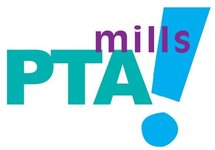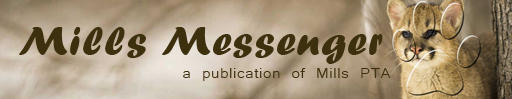## Odom Adopt-A-Family - happening now!

 /* styles */ The holidays should be a time of hope and giving, but for many Odom Elementary families, especially this year, the holidays can be stressful. At Joe Dan Mills Elementary, through the support of our generous Mills families and faculty, we'll make every effort to make the 2021 holiday season a little brighter for those in need. A detailed wishlist from Odom families is below. The official event will take place on Saturday, December 18th. Please make sure to follow our Facebook group for any changes or updates to the wish list. https://bit.ly/millsodom For questions, please contact PTA Community Outreach Chair, May Martin at maymmartin@yahoo.com.
 table div table+table+table+table div table{width:100%;padding:0}table div table+table+table+table div table img{width:96.23%;padding:0;float:none}table div table+table+table+table div table td{width:100%;padding:0 1.88% 18px}/* styles */## 2021 Coats for Kids Coat Drive Competition

 table div table+table+table+table+table+table div table,table.module-5{width:71.7%;float:right;padding:0}table div table+table+table+table+table+table div table a{border:0 none;text-decoration:none}table div table+table+table+table+table+table div table img{width:100%!important;border:0 none;text-decoration:none}table div table+table+table+table+table+table div table td{width:100%;padding:0 0 20px 20px}/* styles */ It's time to bring in those new and gently used coats for the annual Junior League of Austin's Coats for Kids Coat Drive Competition! Mills families have such generous hearts, and we're always overflowing with donations to help keep local families in need warm this winter. There will be a blue donation bin with a sign marked "Donation Bin for Coats for Kids" in the front parking lot. Just drive or walk right up and drop them off, the Community Outreach Committee will take it from there! We welcome new and gently used coats of all colors and sizes including adult-sized coats for teenagers. The coat drive runs from Monday, November 15th through Thursday, December 2nd. Let's show our Mountain Lion spirit by helping us reach our goal of 250 coats (that’s 50 more than last year) and let's win the competition for elementary schools this year! We'll keep you posted here and on the Facebook page with a running tally. Thank you in advance for always showing your compassion for our community.
 table div table+table+table+table+table+table+table div table{width:100%;padding:0}table div table+table+table+table+table+table+table div table img{width:96.23%;padding:0;float:none}table div table+table+table+table+table+table+table div table td{width:100%;padding:0 1.88% 18px}/* styles */table div table+table+table+table+table+table+table+table div table td,table.module-7{width:100%;padding:0}table div table+table+table+table+table+table+table+table div table{width:100%;float:none;margin-left:auto;margin-right:auto;padding:0}table div table+table+table+table+table+table+table+table div table a{border:0 none;text-decoration:none}table div table+table+table+table+table+table+table+table div table img{width:100%!important;border:0 none;text-decoration:none}/* styles */
 table div table+table+table+table+table+table+table+table+table div table{width:100%;padding:0}table div table+table+table+table+table+table+table+table+table div table img{width:96.23%;padding:0;float:none}table div table+table+table+table+table+table+table+table+table div table td{width:100%;padding:0 1.88% 18px}/* styles */## Dirt Club! December 5, 1-4 PM

 /* styles */ Come work off some of that Thanksgiving meal! Our next landscaping outing is planned for Sunday, December 5 from 1-4 PM. Activities may include putting up a new outdoor dry erase board, clearing the fence line along Escarpment, and any touch-up painting we want to do. Come for any amount of time that afternoon and we'll put you to work! Bring some drinking water, gloves, and any pruning loppers if you have them. If you're willing to help out clearing the fences I'd recommend jeans and a long-sleeve shirt (there are thorns). We'll have plenty of thorn-free activities as well. Hope to see you there!
 table div table+table+table+table+table+table+table+table+table+table+table+table div table{width:100%;padding:0}table div table+table+table+table+table+table+table+table+table+table+table+table div table img{width:96.23%;padding:0;float:none}table div table+table+table+table+table+table+table+table+table+table+table+table div table td{width:100%;padding:0 1.88% 18px}/* styles */## Schlitterbahn Waves of Pages Reading Program

 table div table+table+table+table+table+table+table+table+table+table+table+table+table+table div table td,table.module-13{width:100%;padding:0}table div table+table+table+table+table+table+table+table+table+table+table+table+table+table div table{width:100%;float:none;margin-left:auto;margin-right:auto;padding:0}table div table+table+table+table+table+table+table+table+table+table+table+table+table+table div table a{border:0 none;text-decoration:none}table div table+table+table+table+table+table+table+table+table+table+table+table+table+table div table img{width:100%!important;border:0 none;text-decoration:none}/* styles */
 table div table+table+table+table+table+table+table+table+table+table+table+table+table+table+table div table{width:100%;padding:0}table div table+table+table+table+table+table+table+table+table+table+table+table+table+table+table div table img{width:96.23%;padding:0;float:none}table div table+table+table+table+table+table+table+table+table+table+table+table+table+table+table div table td{width:100%;padding:0 1.88% 18px}/* styles */table div table+table+table+table+table+table+table+table+table+table+table+table+table+table+table+table div table td,table.module-15{width:100%;padding:0}table div table+table+table+table+table+table+table+table+table+table+table+table+table+table+table+table div table{width:100%;float:none;margin-left:auto;margin-right:auto;padding:0}table div table+table+table+table+table+table+table+table+table+table+table+table+table+table+table+table div table a{border:0 none;text-decoration:none}table div table+table+table+table+table+table+table+table+table+table+table+table+table+table+table+table div table img{width:100%!important;border:0 none;text-decoration:none}/* styles */
 table div table+table+table+table+table+table+table+table+table+table+table+table+table+table+table+table+table div table{width:100%;padding:0}table div table+table+table+table+table+table+table+table+table+table+table+table+table+table+table+table+table div table img{width:96.23%;padding:0;float:none}table div table+table+table+table+table+table+table+table+table+table+table+table+table+table+table+table+table div table td{width:100%;padding:0 1.88% 18px}/* styles */## Affiliate News

 table div table+table+table+table+table+table+table+table+table+table+table+table+table+table+table+table+table+table+table div table td,table.module-18{width:100%;padding:0}table div table+table+table+table+table+table+table+table+table+table+table+table+table+table+table+table+table+table+table div table{width:100%;float:none;margin-left:auto;margin-right:auto;padding:0}table div table+table+table+table+table+table+table+table+table+table+table+table+table+table+table+table+table+table+table div table a{border:0 none;text-decoration:none}table div table+table+table+table+table+table+table+table+table+table+table+table+table+table+table+table+table+table+table div table img{width:100%!important;border:0 none;text-decoration:none}/* styles */
 table div table+table+table+table+table+table+table+table+table+table+table+table+table+table+table+table+table+table+table+table div table{width:100%;padding:0}table div table+table+table+table+table+table+table+table+table+table+table+table+table+table+table+table+table+table+table+table div table img{width:96.23%;padding:0;float:none}table div table+table+table+table+table+table+table+table+table+table+table+table+table+table+table+table+table+table+table+table div table td{width:100%;padding:0 1.88% 18px}/* styles */## Need to get your message out?

 /* styles */ Submit info to the Mills Messenger. Want to see the Mills Calendar on your Google calendar? Add Mills Calendar to my Google Calendar. Have a friend who isn't getting the Messenger but wants to? Send them this link to sign up: https://madmimi.com/signups/join/7059
 table div table+table+table+table+table+table+table+table+table+table+table+table+table+table+table+table+table+table+table+table+table+table+table div table{width:100%;padding:0}table div table+table+table+table+table+table+table+table+table+table+table+table+table+table+table+table+table+table+table+table+table+table+table div table img{width:96.23%;padding:0;float:none}table div table+table+table+table+table+table+table+table+table+table+table+table+table+table+table+table+table+table+table+table+table+table+table div table td{width:100%;padding:0 1.88% 18px}/* styles *//* styles */ Find recent editions of the Mills Messenger at millspta.org.